mscroggs.co.uk
mscroggs.co.uksubscribe

# Blog

2020-05-15
This is a post I wrote for The Aperiodical's Big Lock-Down Math-Off. You can vote for (or against) me here until 9am on Sunday...
Recently, I came across a surprising fact: if you take any quadrilateral and join the midpoints of its sides, then you will form a parallelogram.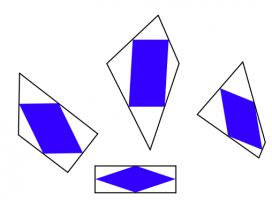The blue quadrilaterals are all parallelograms.
The first thing I thought when I read this was: "oooh, that's neat." The second thing I thought was: "why?" It's not too difficult to show why this is true; you might like to pause here and try to work out why yourself before reading on...
To show why this is true, I started by letting $$\mathbf{a}$$, $$\mathbf{b}$$, $$\mathbf{c}$$ and $$\mathbf{d}$$ be the position vectors of the vertices of our quadrilateral. The position vectors of the midpoints of the edges are the averages of the position vectors of the two ends of the edge, as shown below.
We want to show that the orange and blue vectors below are equal (as this is true of opposite sides of a parallelogram).
We can work these vectors out: the orange vector is$$\frac{\mathbf{d}+\mathbf{a}}2-\frac{\mathbf{a}+\mathbf{b}}2=\frac{\mathbf{d}-\mathbf{b}}2,$$ and the blue vector is$$\frac{\mathbf{c}+\mathbf{d}}2-\frac{\mathbf{b}+\mathbf{c}}2=\frac{\mathbf{d}-\mathbf{b}}2.$$
In the same way, we can show that the other two vectors that make up the inner quadrilateral are equal, and so the inner quadrilateral is a parallelogram.

### Going backwards

Even though I now saw why the surprising fact was true, my wondering was not over. I started to think about going backwards.
It's easy to see that if the outer quadrilateral is a square, then the inner quadrilateral will also be a square.If the outer quadrilateral is a square, then the inner quadrilateral is also a square.
It's less obvious if the reverse is true: if the inner quadrilateral is a square, must the outer quadrilateral also be a square? At first, I thought this felt likely to be true, but after a bit of playing around, I found that there are many non-square quadrilaterals whose inner quadrilaterals are squares. Here are a few: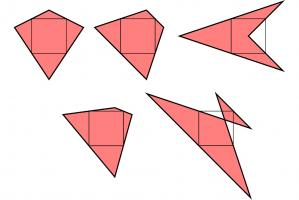A kite, a trapezium, a delta kite, an irregular quadrilateral and a cross-quadrilateral whose innner quadrilaterals are all a square.
There are in fact infinitely many quadrilaterals whose inner quadrilateral is a square. You can explore them in this Geogebra applet by dragging around the blue point:
As you drag the point around, you may notice that you can't get the outer quadrilateral to be a non-square rectangle (or even a non-square parallelogram). I'll leave you to figure out why not...

### Similar posts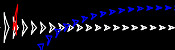Mathsteroids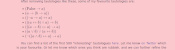Interesting tautologies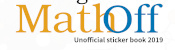Big Internet Math-Off stickers 2019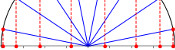Runge's Phenomenon

Comments in green were written by me. Comments in blue were not written by me.

Allowed HTML tags: <br> <a> <small> <b> <i> <s> <sup> <sub> <u> <spoiler> <ul> <ol> <li>
To prove you are not a spam bot, please type "integer" in the box below (case sensitive):
2020-05-03
This is a post I wrote for The Aperiodical's Big Lock-Down Math-Off. You can vote for (or against) me here until 9am on Tuesday...
A few years ago, I made @mathslogicbot, a Twitter bot that tweets logical tautologies.
The statements that @mathslogicbot tweets are made up of variables (a to z) that can be either true or false, and the logical symbols $$\lnot$$ (not), $$\land$$ (and), $$\lor$$ (or), $$\rightarrow$$ (implies), and $$\leftrightarrow$$ (if and only if), as well as brackets. A tautology is a statement that is always true, whatever values are assigned to the variables involved.
To get an idea of how to interpret @mathslogicbot's statements, let's have a look at a few tautologies:
$$( a \rightarrow a )$$. This says "a implies a", or in other words "if a is true, then a is true". Hopefully everyone agrees that this is an always-true statement.
$$( a \lor \lnot a )$$. This says "a or not a": either a is true, or a is not true
$$(a\leftrightarrow a)$$. This says "a if and only if a".
$$\lnot ( a \land \lnot a )$$. This says "not (a and not a)": a and not a cannot both be true.
$$( \lnot a \lor \lnot \lnot a )$$. I'll leave you to think about what this one means.
(Of course, not all statements are tautologies. The statement $$(b\land a)$$, for example, is not a tautology as is can be true or false depending on the values of $$a$$ and $$b$$.)
While looking through @mathslogicbot's tweets, I noticed that a few of them are interesting, but most are downright rubbish. This got me thinking: could I get rid of the bad tautologies like these, and make a list of just the "interesting" tautologies. To do this, we first need to think of different ways tautologies can be bad.
Looking at tautologies the @mathslogicbot has tweeted, I decided to exclude:
• tautologies like $$(a\rightarrow\lnot\lnot\lnot\lnot a)$$ that contain more than one $$\lnot$$ in a row.
• tautologies like $$((a\lor\lnot a)\lor b)$$ that contain a shorter tautology. Instead, tautologies like $$(\text{True}\lor b)$$ should be considered.
• tautologies like $$((a\land\lnot a)\rightarrow b)$$ that contain a shorter contradiction (the opposite of a tautology). Instead, tautologies like $$(\text{False}\rightarrow b)$$ should be considered.
• tautologies like $$(\text{True}\lor\lnot\text{True})$$ or $$((b\land a)\lor\lnot(b\land a)$$ that are another tautology (in this case $$(a\lor\lnot a)$$) with a variable replaced with something else.
• tautologies containing substatements like $$(a\land a)$$, $$(a\lor a)$$ or $$(\text{True}\land a)$$ that are equivalent to just writing $$a$$.
• tautologies that contain a $$\rightarrow$$ that could be replaced with a $$\leftrightarrow$$, because it's more interesting if the implication goes both ways.
• tautologies containing substatements like $$(\lnot a\lor\lnot b)$$ or $$(\lnot a\land\lnot b)$$ that could be replaced with similar terms (in these cases $$(a\land b)$$ and $$(a\lor b)$$ respectively) without the $$\lnot$$s.
• tautologies that are repeats of each other with the order changed. For example, only one of $$(a\lor\lnot a)$$ and $$(\lnot a\lor a)$$ should be included.
After removing tautologies like these, some of my favourite tautologies are:
• $$( \text{False} \rightarrow a )$$
• $$( a \rightarrow ( b \rightarrow a ) )$$
• $$( ( \lnot a \rightarrow a ) \leftrightarrow a )$$
• $$( ( ( a \leftrightarrow b ) \land a ) \rightarrow b )$$
• $$( ( ( a \rightarrow b ) \leftrightarrow a ) \rightarrow a )$$
• $$( ( a \lor b ) \lor ( a \leftrightarrow b ) )$$
• $$( \lnot ( ( a \land b ) \leftrightarrow a ) \rightarrow a )$$
• $$( ( \lnot a \rightarrow b ) \leftrightarrow ( \lnot b \rightarrow a ) )$$
You can find a list of the first 500 "interesting" tautologues here. Let me know on Twitter which is your favourite. Or let me know which ones you think are rubbish, and we can further refine the list...

### Similar posts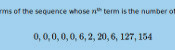Logical contradictions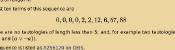Logic bot, pt. 2Logic bot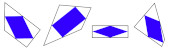A surprising fact about quadrilaterals

Comments in green were written by me. Comments in blue were not written by me.

Allowed HTML tags: <br> <a> <small> <b> <i> <s> <sup> <sub> <u> <spoiler> <ul> <ol> <li>
To prove you are not a spam bot, please type "s" then "e" then "g" then "m" then "e" then "n" then "t" in the box below (case sensitive):
2020-03-31
Recently, you've probably seen a lot of graphs that look like this:
The graph above shows something that is growing exponentially: its equation is $$y=kr^x$$, for some constants $$k$$ and $$r$$. The value of the constant $$r$$ is very important, as it tells you how quickly the value is going to grow. Using a graph of some data, it is difficult to get an anywhere-near-accurate approximation of $$r$$.
The following plot shows three different exponentials. It's very difficult to say anything about them except that they grow very quickly above around $$x=15$$.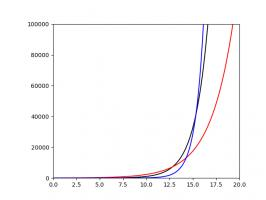$$y=2^x$$, $$y=40\times 1.5^x$$, and $$y=0.002\times3^x$$
It would be nice if we could plot these in a way that their important properties—such as the value of the ratio $$r$$—were more clearly evident from the graph. To do this, we start by taking the log of both sides of the equation:
$$\log y=\log(kr^x)$$
Using the laws of logs, this simplifies to:
$$\log y=\log k+x\log r$$
This is now the equation of a straight line, $$\hat{y}=m\hat{x}+c$$, with $$\hat{y}=\log y$$, $$\hat{x}=x$$, $$m=\log r$$ and $$c=\log k$$. So if we plot $$x$$ against $$\log y$$, we should get a straight line with gradient $$\log r$$. If we plot the same three exponentials as above using a log-scaled $$y$$-axis, we get:$$y=2^x$$, $$y=40\times 1.5^x$$, and $$y=0.002\times3^x$$ with a log-scaled $$y$$-axis
From this picture alone, it is very clear that the blue exponential has the largest value of $$r$$, and we could quickly work out a decent approximation of this value by calculating 10 (or the base of the log used if using a different log) to the power of the gradient.

### Log-log plots

Exponential growth isn't the only situation where scaling the axes is beneficial. In my research in finite and boundary element methods, it is common that the error of the solution $$e$$ is given in terms of a grid parameter $$h$$ by a polynomial of the form $$e=ah^k$$, for some constants $$a$$ and $$k$$.
We are often interested in the value of the power $$k$$. If we plot $$e$$ against $$h$$, it's once again difficult to judge the value of $$k$$ from the graph alone. The following graph shows three polynomials.$$y=x^2$$, $$y=x^{1.5}$$, and $$y=0.5x^3$$
Once again is is difficult to judge any of the important properties of these. To improve this, we once again begin by taking the log of each side of the equation:
$$\log e=\log (ah^k)$$
Applying the laws of logs this time gives:
$$\log e=\log a+k\log h$$
This is now the equation of a straight line, $$\hat{y}=m\hat{x}+c$$, with $$\hat{y}=\log e$$, $$\hat{x}=\log h$$, $$m=k$$ and $$c=\log a$$. So if we plot $$\log x$$ against $$\log y$$, we should get a straight line with gradient $$k$$.
Doing this for the same three curves as above gives the following plot.$$y=x^2$$, $$y=x^{1.5}$$, and $$y=0.5x^3$$ with log-scaled $$x$$- and $$y$$-axes
It is easy to see that the blue line has the highest value of $$k$$ (as it has the highest gradient, and we could get a decent approximation of this value by finding the line's gradient.

As well as making it easier to get good approximations of important parameters, making curves into straight lines in this way also makes it easier to plot the trend of real data. Drawing accurate exponentials and polynomials is hard at the best of times; and real data will not exactly follow the curve, so drawing an exponential or quadratic of best fit will be an even harder task. By scaling the axes first though, this task simplifies to drawing a straight line through the data; this is much easier.
So next time you're struggling with an awkward curve, why not try turning it into a straight line first.

### Similar posts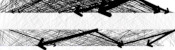Visualising MENACE's learningWorld Cup stickers 2018, pt. 2A surprising fact about quadrilateralsInteresting tautologies

Comments in green were written by me. Comments in blue were not written by me.

Allowed HTML tags: <br> <a> <small> <b> <i> <s> <sup> <sub> <u> <spoiler> <ul> <ol> <li>
To prove you are not a spam bot, please type "ratio" in the box below (case sensitive):
2020-02-17
 This is the sixth post in a series of posts about my PhD thesis.
Before we move on to some concluding remarks and notes about possible future work, I must take this opportunity to thank my co-supervisors Timo Betcke and Erik Burman, as without their help, support, and patience, this work would never have happened.

### Future work

There are of course many things related to the work in my thesis that could be worked on in the future by me or others.
In the thesis, we presented the analysis of the weak imposition of Dirichlet, Neumann, mixed Dirichlet–Neumann, Robin, and Signorini boundary conditions on Laplace's equation; and Dirichlet, and mixed Dirichlet–Neumann conditions on the Helmholtz equation. One area of future work would be to extend this analysis to other conditions, such as the imposition of Robin conditions on the Helmholtz equation. It would also be of great interest to extend the method to other problems, such as Maxwell's equations. For Maxwell's equations, it looks like the analysis will be significantly more difficult.
In the problems in later chapters, in particular chapter 4, the ill-conditioning of the matrices obtained from the method led to slow or even inaccurate solutions. It would be interesting to look into alternative preconditioning methods for these problems as a way to improve the conditioning of these matrices. Developing these preconditioners appears to be very important for Maxwell's equations: general the matrices involved when solving Maxwell's equations tend to be very badly ill-conditioned, and in the few experiments I ran to try out the weak imposition of boundary conditions on Maxwell's equations, I was unable to get a good solution due to this.

If you are a undergraduate or master's student and are interested in working on similar stuff to me, then you could look into doing a PhD with Timo and/or Erik (my supervisors). There are also many other people around working on similar stuff, including:
There are of course many, many more people working on this, and this list is in no way exhaustive. But hopefully this list can be a useful starting point for anyone interested in studying this area of maths.
Previous post in series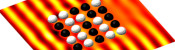PhD thesis, chapter 5
This is the sixth post in a series of posts about my PhD thesis.

### Similar postsPhD thesis, chapter 5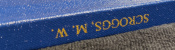PhD thesis, chapter 4PhD thesis, chapter 3PhD thesis, chapter 2

Comments in green were written by me. Comments in blue were not written by me.

Allowed HTML tags: <br> <a> <small> <b> <i> <s> <sup> <sub> <u> <spoiler> <ul> <ol> <li>
To prove you are not a spam bot, please type "rotcev" backwards in the box below (case sensitive):
2020-02-16
 This is the fifth post in a series of posts about my PhD thesis.
In the fifth and final chapter of my thesis, we look at how boundary conditions can be weakly imposed on the Helmholtz equation.

### Analysis

As in chapter 4, we must adapt the analysis of chapter 3 to apply to Helmholtz problems. The boundary operators for the Helmholtz equation satisfy less strong conditions than the operators for Laplace's equation (for Laplace's equation, the operators satisfy a condition called coercivity; for Helmholtz, the operators satisfy a weaker condition called Gårding's inequality), making proving results about Helmholtz problem harder.
After some work, we are able to prove an a priori error bound (with $$a=\tfrac32$$ for the spaces we use):
$$\left\|u-u_h\right\|\leqslant ch^{a}\left\|u\right\|$$

### Numerical results

As in the previous chapters, we use Bempp to show that computations with this method match the theory.The error of our approximate solutions of a Dirichlet (left) and mixed Dirichlet–Neumann problems in the exterior of a sphere with meshes with different values of $$h$$. The dashed lines show order $$\tfrac32$$ convergence.

### Wave scattering

Boundary element methods are often used to solve Helmholtz wave scattering problems. These are problems in which a sound wave is travelling though a medium (eg the air), then hits an object: you want to know what the sound wave that scatters off the object looks like.
If there are multiple objects that the wave is scattering off, the boundary element method formulation can get quite complicated. When using weak imposition, the formulation is simpler: this one advantage of this method.
The following diagram shows a sound wave scattering off a mixure of sound-hard and sound-soft spheres. Sound-hard objects reflect sound well, while sound-soft objects absorb it well.A sound wave scattering off a mixture of sound-hard (white) and sound-soft (black) spheres.
If you are trying to design something with particular properties—for example, a barrier that absorbs sound—you may want to solve lots of wave scattering problems on an object on some objects with various values taken for their reflective properties. This type of problem is often called an inverse problem.
For this type of problem, weakly imposing boundary conditions has advantages: the discretisation of the Calderón projector can be reused for each problem, and only the terms due to the weakly imposed boundary conditions need to be recalculated. This is an advantages as the boundary condition terms are much less expensive (ie they use much less time and memory) to calculate than the Calderón term that is reused.

This concludes chapter 5, the final chapter of my thesis. Why not celebrate reaching the end by cracking open the following figure before reading the concluding blog post.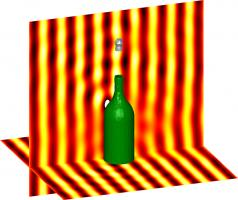An acoustic wave scattering off a sound-hard champagne bottle and a sound-soft cork.
Previous post in seriesPhD thesis, chapter 4
This is the fifth post in a series of posts about my PhD thesis.
Next post in seriesPhD thesis, chapter ∞

### Similar postsPhD thesis, chapter 4PhD thesis, chapter 3PhD thesis, chapter ∞PhD thesis, chapter 2

Comments in green were written by me. Comments in blue were not written by me.